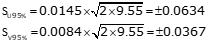### Problem (4)

The following traverse was adjusted by least squares to determine the coordinates of points G and H.Final coordinates of G and H are:

 Point North East G 4,958.913 917.702 H 5,067.094 1,252.311

Matrices from the final iteration of the adjustment are:#### Compute:

(a) Expected uncertainties of the adjusted coordinates.

(c) Parameters of the standard and 95% CI error ellipsii at each adjusted point.

### Solution

(a) Expected uncertainties of the adjusted coordinates.

Residuals are computed from [V] = [CU] - [K]

Since the [U] elements are all zero, [V] = -[K]Add the [V] matrix elements to the corresponding field measurements to get the adjusted observations.(c) Standard and 95% CI error eliipsii

Point GPoint HError Ellipse Summary

 Std 95% CI Point AzU a b a b G 9°32'48" 0.0075 0.0066 0.033 0.029 H 12°57'42" 0.0145 0.0084 0.0634 0.037